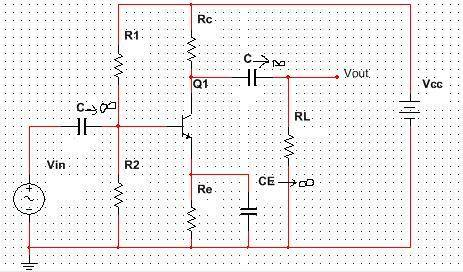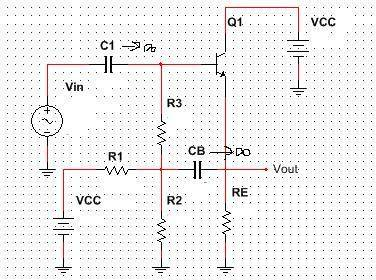# BJT current gain problem

Hi!
Calculate voltage gain Av and current gain Ai for circuit on scheme .I don't know how to calculate Ai. I got that Av=-gm*rce*Rc*Rl/(Rc*Rl+rce*(Rc+Rl)) and it matches with solution given in my textbook.
I know that Ai=Iout/Iin but which current is Iout? Ai should be gm*rce/((rce+Rc*Rl/(Rc+Rl))*(1/R1+1/R2+1/rpi)).

NascentOxygen
Staff Emeritus
Iout is the current through the load, RL.

•1 person
It seemed to me also that Iout is current through Rl but when I calculate Iout/Iin it doesn't match with solution in textbook :(

The Electrician
Gold Member
The current that corresponds to gm*rce/((rce+Rc*Rl/(Rc+Rl))*(1/R1+1/R2+1/rpi)) is the total collector current; the sum of the currents in Rc and RL.

•1 person
NascentOxygen
Staff Emeritus
It seemed to me also that Iout is current through Rl but when I calculate Iout/Iin it doesn't match with solution in textbook :(
Well, the current through RL definitely should be Iout.

However, you could imagine an additional load in parallel with RL, with Iout going into that additional load. But this is just equivalent to making RL smaller.

@The Electrician
Does Iout depend of circuit configuration or it is always Ic? What would be Iout for this circuit:?

The Electrician
Gold Member
I would say that what Iout is, depends on the intention of the problem originator.

Iout for the circuit in post #1 is the sum of the currents in Rc and RL because that's what gives the answer from your book.

Iout for the circuit you show in post #6 could be the total current out of the emitter of Q1, or it could be the current only in a load connected to Vout.

Here is current gain for circuit in my previous post:
Ai=(1+gm*R3pi)*rce/(R12E+rce), where R12E=R1||R2||RE, R3pi=R3||rpi.
Really tricky for me...

The Electrician
Gold Member
What is the definition of current gain you have used? Is it the total emitter current, or something else?

The Electrician
Gold Member
Here is current gain for circuit in my previous post:
Ai=(1+gm*R3pi)*rce/(R12E+rce), where R12E=R1||R2||RE, R3pi=R3||rpi.
Really tricky for me...

If I take Iout to be the sum of the currents in R1, R2 and RE then I get your result. Is that what you used for Iout?

What is the definition of current gain you have used? Is it the total emitter current, or something else?
We did in class basic BJT amplifier configurations (common base, common collector and common emittor) and Iout is differently defined for every configuration. Maybe my task is incomplete, it should be emphasized what Iout is I think.
Thanks for help guys!